# MQL5 Indicators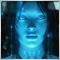27

Hey guys,

I am reprogramming my MQL4 code to MQL5. I do have a question about the indicator change.

So in MQL4 it simply looks something like:

```void OnTick()
{
double movingAverage = iMA(NULL,PERIOD_CURRENT,13,0,MODE_EMA,PRICE_CLOSE,0);
}```

So the variable gets updated every tick.

But in MQL5 we have to I guess overcomplicate things.

```//GLOBAL DATA

double moving_average;
double movingAverage[];

//+---------------------------------------------------------------------------------------------------------+
//|                                                INITIALIZATION                                           |
//+---------------------------------------------------------------------------------------------------------+
int OnInit()
{
moving_average = iMA(NULL,PERIOD_CURRENT,20,0,MODE_EMA,PRICE_CLOSE);
CopyBuffer(moving_average,0,0,100,movingAverage)
ArraySetAsSeries(movingAverage,true);

}

//We skip OnDeinit here

//+---------------------------------------------------------------------------------------------------------+
//|                                               EXECUTE ON TICK                                           |
//+---------------------------------------------------------------------------------------------------------+
void OnTick()
{

double LastMovingAverage = movingAverage;

```

So my question is does the moving average prices get updated like it does in my MQL 4 code? Or Do I have to shift things from OnInit to the OnTick function?  Because OnInit only executes one time...

Would appreciate help with this!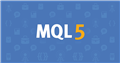Documentation on MQL5: Constants, Enumerations and Structures / Indicator Constants / Price Constants
• www.mql5.com
Calculations of technical indicators require price values and/or values of volumes, on which calculations will be performed. There are 7 predefined identifiers from the ENUM_APPLIED_PRICE enumeration, used to specify the desired price base for calculations. If a technical indicator uses for calculations price data, type of which is set by...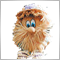Moderator
192899

Seantjuh1991 :

Hey guys,

I am reprogramming my MQL4 code to MQL5. I do have a question about the indicator change.

So in MQL4 it simply looks something like:

So the variable gets updated every tick.

But in MQL5 we have to I guess overcomplicate things.

So my question is does the moving average prices get updated like it does in my MQL 4 code? Or Do I have to shift things from OnInit to the OnTick function?  Because OnInit only executes one time...

Would appreciate help with this!

In OnInit, you need to create an indicator handle.

In OnTick you need to use copying.

### Creating an iMA indicator handle, getting indicator values

(code: iMA Values on a Chart)

The main rule: the indicator handle needs to be received once (it is optimal to do this in the OnInit function).

Input parameters and the variable in which the indicator handle is stored:

```//+------------------------------------------------------------------+
//|                                        iMA Values on a Chart.mq5 |
//+------------------------------------------------------------------+
#property version   "1.00"
//--- input parameters
input int                  Inp_MA_ma_period     = 12;          // MA: averaging period
input int                  Inp_MA_ma_shift      = 5;           // MA: horizontal shift
input ENUM_MA_METHOD       Inp_MA_ma_method     = MODE_SMA;    // MA: smoothing type
input ENUM_APPLIED_PRICE   Inp_MA_applied_price = PRICE_CLOSE; // MA: type of price
//---
int    handle_iMA;                           // variable for storing the handle of the iMA indicator
//+------------------------------------------------------------------+
//| Expert initialization function                                   |```

Creating a handle (checking the result)

```//+------------------------------------------------------------------+
//| Expert initialization function                                   |
//+------------------------------------------------------------------+
int OnInit()
{
//--- create handle of the indicator iMA
handle_iMA=iMA(Symbol(),Period(),Inp_MA_ma_period,Inp_MA_ma_shift,
Inp_MA_ma_method,Inp_MA_applied_price);
//--- if the handle is not created
if(handle_iMA==INVALID_HANDLE)
{
//--- tell about the failure and output the error code
PrintFormat("Failed to create handle of the iMA indicator for the symbol %s/%s, error code %d",
Symbol(),
EnumToString(Period()),
GetLastError());
//--- the indicator is stopped early
return(INIT_FAILED);
}
//---
return(INIT_SUCCEEDED);
}```

Getting values (I use the iGetArray generic function). Please note: for the array "array_ma" I use "ArraySetAsSeries" - in this case, in the “array_ma” array, the element with the index “0” corresponds to the rightmost bar on the chart (bar # 0)

```//+------------------------------------------------------------------+
//| Expert tick function                                             |
//+------------------------------------------------------------------+
void OnTick()
{
//---
double array_ma[];
ArraySetAsSeries(array_ma,true);
int start_pos=0,count=3;
if(!iGetArray(handle_iMA,0,start_pos,count,array_ma))
return;

string text="";
for(int i=0; i<count; i++)
text=text+IntegerToString(i)+": "+DoubleToString(array_ma[i],Digits()+1)+"\n";
//---
Comment(text);
}```

The result of work: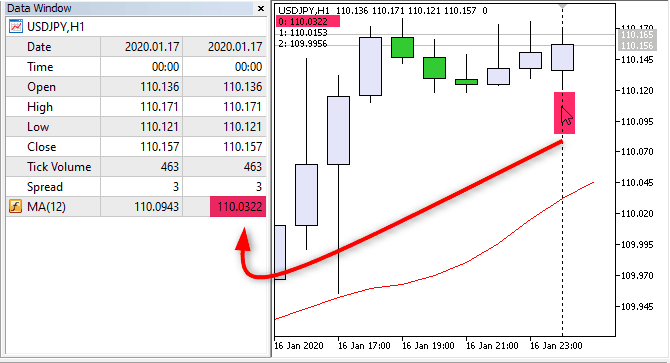Pic. 1. Get value on bar #0, #1, #2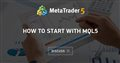• 2020.03.05
• www.mql5.com
This thread discusses MQL5 code examples. There will be examples of how to get data from indicators, how to program advisors...27